# Civil Engineering - Theory of Structures

Exercise : Theory of Structures - Section 1
41.
A shaft is subjected to bending moment M and a torque T simultaneously. The ratio of the maximum bending stress to maximum shear stress developed in the shaft, is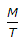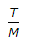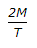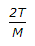Explanation:
No answer description is available. Let's discuss.

42.
The ratio of the deflections of the free end of a cantilever due to an isolated load at l/3rd and 2/3rd of the span, is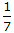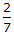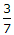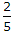Explanation:
No answer description is available. Let's discuss.

43.
If Q is load factor, S is shape factor and F is factor of safety in elastic design, the following:
Q = S + F
Q = S - F
Q = F - S
Q = S x F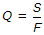Explanation:
No answer description is available. Let's discuss.

44.
In plastic analysis, the shape factor for a triangular section, is
1.5
1.34
2.34
2.5